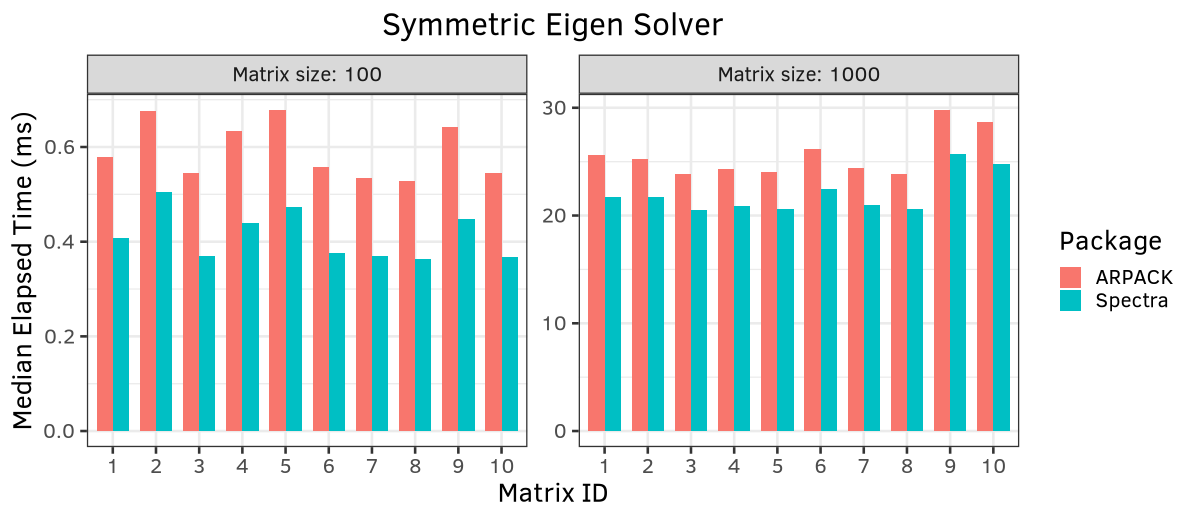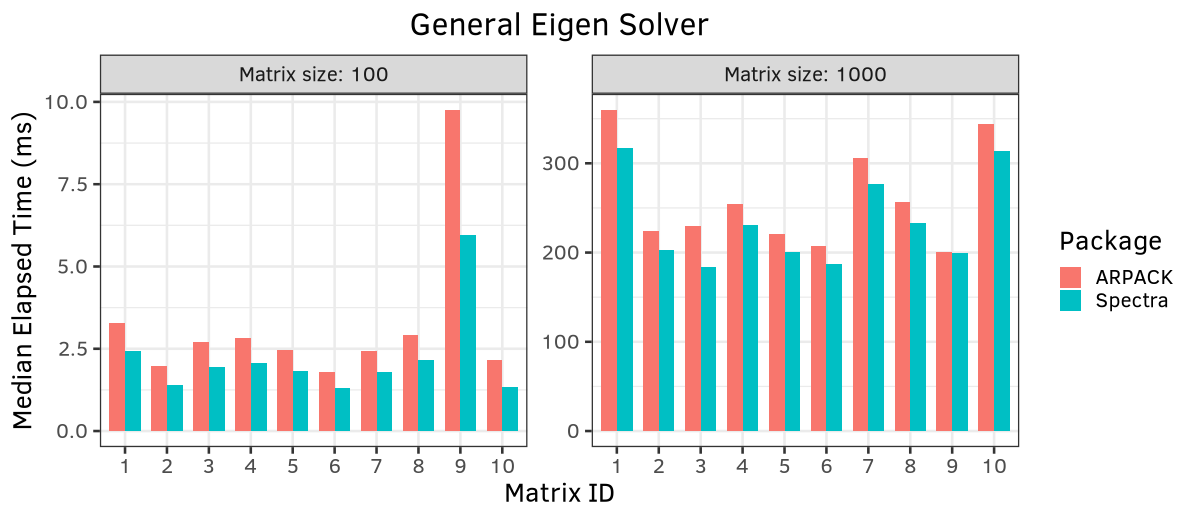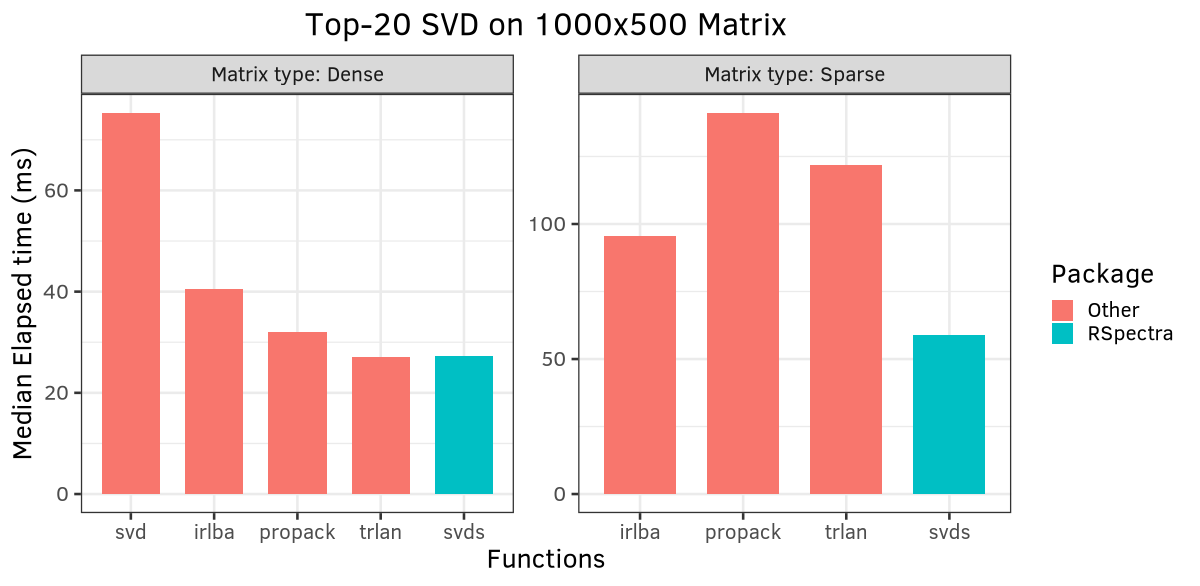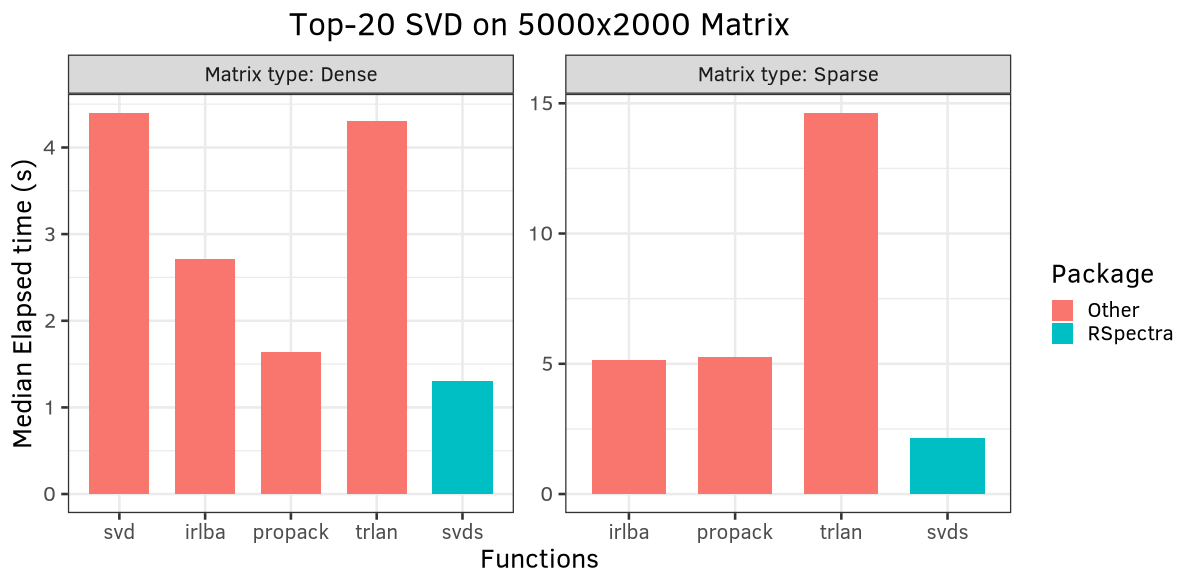# Performance

This page shows some benchmark results of Spectra compared with other similar libraries, based on the following environment setting:

#### Hardware

• CPU: Intel Core i7-11800H 2.30GHz x 16
• Memory: 32GB

#### Software

• OS: Solus 4.2 64-bit
• Compiler: Clang 11.1.0 with flag `clang++ -Wall -O2 -march=native`
• Spectra: v1.0.0
• BLAS: OpenBLAS 0.3.15, single threaded
• ARPACK: ARPACK-NG 3.7.0
• R: version 4.1.0
• R packages
• RSpectra 0.16-0
• svd 0.5
• irlba 2.3.3
• microbenchmark 1.4.7

### Comparison with ARPACK

Spectra is designed to be comparable to or even faster than ARPACK. The benchmark directory in Spectra’s source code contains a program to benchmark both ARPACK and Spectra on some simulated matrices.

Below shows the comparison results:The symmetric eigen solver by Spectra is uniformly faster than ARPACK in all the tested cases, and general eigen solver is almost identical to ARPACK.

### Comparison with SVD solvers

The R interface of Spectra, RSpectra, is compared with other SVD solvers in R.The figures are generated using the following R code:

``````library(Matrix)
library(RSpectra)
library(svd)
library(irlba)
library(microbenchmark)
library(dplyr)
library(ggplot2)

n = 1000
p = 500
nu = nv = k = 20

set.seed(123)
x = matrix(rnorm(n * p), n)
x[sample(n * p, floor(n * p / 2))] = 0
xsp = as(x, "dgCMatrix")

## For svd package
f = function(v) as.numeric(xsp %*% v)
tf = function(v) as.numeric(crossprod(xsp, v))
extx = extmat(f, tf, nrow(xsp), ncol(xsp))

res = microbenchmark(
"svd"             = svd(x, nu, nv),
"svds"            = svds(x, k, nu, nv, opts = list(tol = 1e-8)),
"propack"         = propack.svd(x, k, opts = list(tol = 1e-8)),
"trlan"           = trlan.svd(x, k, opts = list(tol = 1e-8)),
"irlba"           = irlba(x, nu, nv, tol = 1e-8),
"svds[sparse]"    = svds(xsp, k, nu, nv, opts = list(tol = 1e-8)),
"propack[sparse]" = propack.svd(extx, k, opts = list(tol = 1e-8)),
"trlan[sparse]"   = trlan.svd(extx, k, opts = list(tol = 1e-8)),
"irlba[sparse]"   = irlba(xsp, nu, nv, tol = 1e-8),
times = 10
)

dat = as.data.frame(res)
dat\$type = ifelse(grepl("sparse", dat\$expr), "Matrix type: Sparse",
"Matrix type: Dense")
dat\$expr = factor(gsub("\\[sparse\\]", "", dat\$expr),
levels = c("svd", "irlba", "propack", "trlan", "svds"))
dat = dat %>% group_by(expr, type) %>% summarize(medtime = median(time) / 1e6)
dat\$Package = ifelse(grepl("svds", dat\$expr), "RSpectra", "Other")

ggplot(dat, aes(x = expr, y = medtime)) +
facet_wrap(~ type, scales = "free", ncol = 2) +
geom_bar(aes(fill = Package), stat = "identity", width = 0.7) +
scale_y_continuous("Median Elapsed time (ms)") +
scale_x_discrete("Functions") +
ggtitle(sprintf("Top-%d SVD on %dx%d Matrix", k, n, p)) +
theme_bw(base_size = 20) +
theme(plot.title = element_text(hjust = 0.5))
``````